# Vector group

(diff) ← Older revision | Latest revision (diff) | Newer revision → (diff)
A partially ordered group which is imbeddable in a complete direct product of totally ordered groups (cf. Totally ordered group). A groupis a vector group if and only if its partial order is an intersection of total orders on. A partially ordered group will be a vector group if and only if its semi-groupof positive elements satisfies the following condition: For any finite collection of elements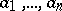of,where this intersection is taken over all combinations of signs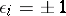, while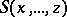denotes the smallest invariant sub-semi-group ofcontaining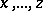. An orderable groupis a vector group if and only if for anyit follows from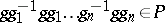that.